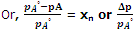Explain solid in liquid solutions.

The French chemist Francois Marie Raoult (1886) carried out a series of experiments to study the vapour pressure of a number of binary solutions. On the basis of the results of the experiments, he proposed a generalization called Raoult's law which states that,

The vapour pressure of a solution containing non-volatile solute is directly proportional to the mole fraction of the solvent.

In case of solution containing two components A (volatile solvent) and B (non-volatile solute) the vapour pressure of solution is given as

[Vapour pressure of solution] = [vapour pressure of solvent in solution (pA) ∝ [mole fraction of solvent (xA)]

Or pA ∝ xA

Or, pA = kxA

Where k is proportionality constant.

For pure liquid, xA = 1 then k becomes equal to be vapour pressure of the pure solvent which is denoted by pA°.

Thus, p= pA°x                                           (i)

Or psolution × mole fraction of solvent.

For solutions obeying Raoult's law at all concentrations its vapour pressure would vary linearly from zero to the vapour pressure of pure solvent.

If mole fraction of solute is sB, then

xA + xB = 1 or xA = 1 - xB                             (ii)

From eqns. (i) and (ii),

pA = pA°(1 - xB) = pA° - pA°xB

Or, pA° - pA = pA°xB#### Related Questions in Chemistry

• ##### Q :Molar mass what is the equation for

what is the equation for calculating molar mass of non volatile solute

• ##### Q :Relationship between Pressure and

The pressure-temperature relation for solid-vapor or liquid vapor equilibrium is expressed by the Clausis-Clapeyron equation.We now obtain an expression for the pressure-temperature dependence of the state of equilibrium between two phases. To be specific,

• ##### Q :Macromolecules what are condensation

what are condensation polymerization give in with 2 examples

• ##### Q :Concentration of an aqueous solution

Give me answer of this question. The concentration of an aqueous solution of 0.01M CH3OH solution is very nearly equal to which of the following : (a) 0.01%CH3OH (b) 0.1%CH3OH (c) xCH3OH= 0.01 (d) 0.99MH2O (

• ##### Q :Production of alcoholic drinks give all

give all physical aspects in the production of alcohol

• ##### Q :The three facts on the evaporation

Describe briefly the three facts on the evaporation?

• ##### Q :Mole fraction Give me answer of

Give me answer of following question. The sum of the mole fraction of the components of a solution is : (a) 0 (b) 1 (c) 2 (d) 4.

• ##### Q :Organic and inorganic chemistry Write

Write down a short note on the differences between the organic and inorganic chemistry?

• ##### Q :IUPAC name of the benzene Write a short

Write a short note on the IUPAC name of the benzene?

• ##### Q :Molarity of cane sugar solution 171 g

171 g of cane sugar (C12H22O11)  is dissolved in one litre of water. Find the molarity of the solution: (i) 2.0 M (ii) 1.0 M (iii) 0.5 M (iv) 0.25 M Choose the right answer from above.